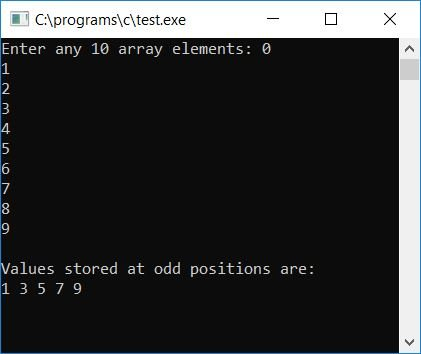# C Program to Print Array Elements Present in Odd Position

In this article, we will learn how to create a program in C that will read array elements and then print all the array elements present at odd positions (index-wise). The question is: write a program in C to read 10 values from an integer array and print all values stored at the odd position. Here is its answer:

```#include<stdio.h>
#include<conio.h>
int main()
{
int arr, i;
printf("Enter any 10 array elements: ");
for(i=0; i<10; i++)
scanf("%d", &arr[i]);
printf("\nValues stored at odd positions are:\n");
for(i=0; i<10; i++)
{
if(i%2!=0)
printf("%d ", arr[i]);
}
getch();
return 0;
}```

The program was written in the Code::Blocks IDE; therefore, after a successful build and run, this is the first snapshot of the sample run:Supply any 10 numbers or elements for the array and press the ENTER key to see the value stored at the odd index position:#### Program Explained

• Receive any 10 numbers as 10 array elements.
• Create a for loop starts from the first number (present at index number 0) to the last number (present at index number 9).
• Check whether the current index number is an odd number or not.
• If it is, then print the value stored at that index number.

### Allow the user to define the array size

Now let's modify the above program to allow the user to decide the length of the array:

```#include<stdio.h>
#include<conio.h>
int main()
{
int arr, i, limit;
printf("How many elements you want to store inside the array: ");
scanf("%d", &limit);
printf("Enter any %d array elements: ", limit);
for(i=0; i<limit; i++)
scanf("%d", &arr[i]);
printf("\nValues stored at odd position (with array and its index) are:\n");
for(i=0; i<limit; i++)
{
if(i%2!=0)
printf("arr[%d] = %d\n", i, arr[i]);
}
getch();
return 0;
}```

Here is the final snapshot of the sample run:The following are some of the main steps in the above program:

• Receive the length of the array, say 20.
• Receive 20 numbers as 20 array elements.
• Follow the same procedure as told in the above program's steps.
• That is to find and print the value present at the odd index number.

C Quiz

« Previous Program Next Program »